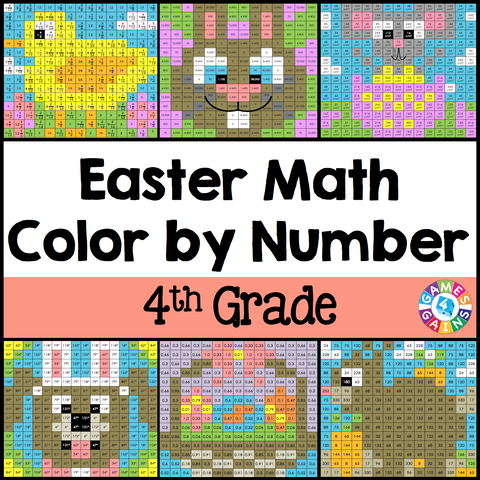## Easter Math Color-by-Number - 4th Grade

• \$480

Easter Math Color-by-Number set comes with 6 Easter Math color-by-number activities for reviewing 4th grade math skills. This Easter Math math set is perfect to use in centers, in small groups, or with the whole class!

Here are the skills covered by these Easter Math math color-by-number activities:

• Solving multiplicative comparison problems with multiples of 10
• Multiplying proper fractions by whole numbers
• Writing decimals from decimal models
• Converting customary lengths from the larger unit to the smaller unit (yards, feet, inches)
• Calculating area
• Determining the missing angle measurement

Included with this Easter Math Math Color-by-Number set:
Instructions
6 color by number worksheets
6 student problem worksheets